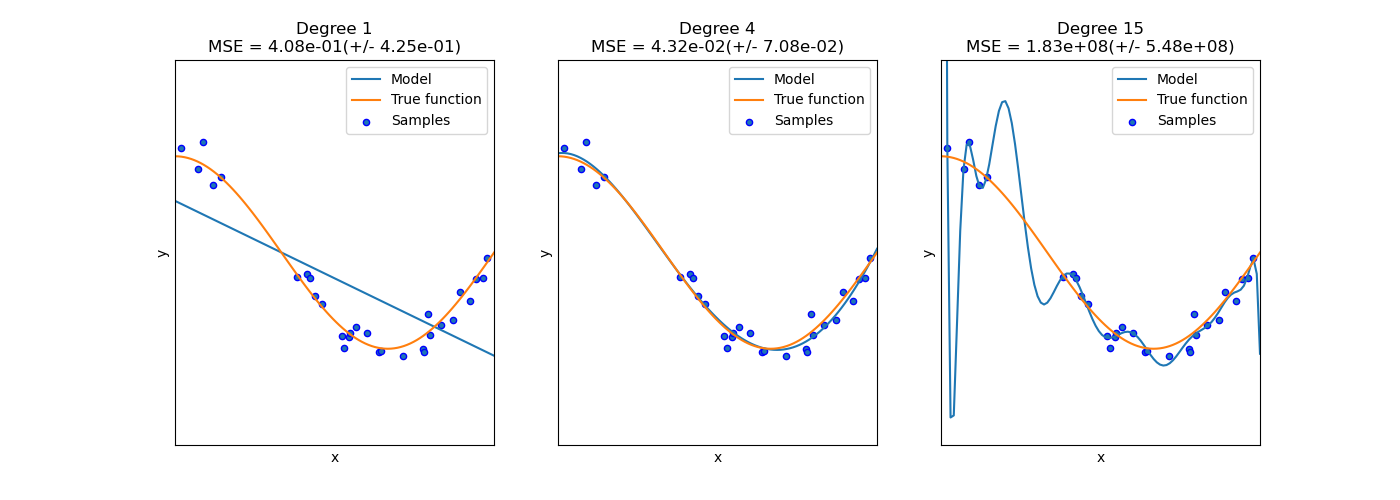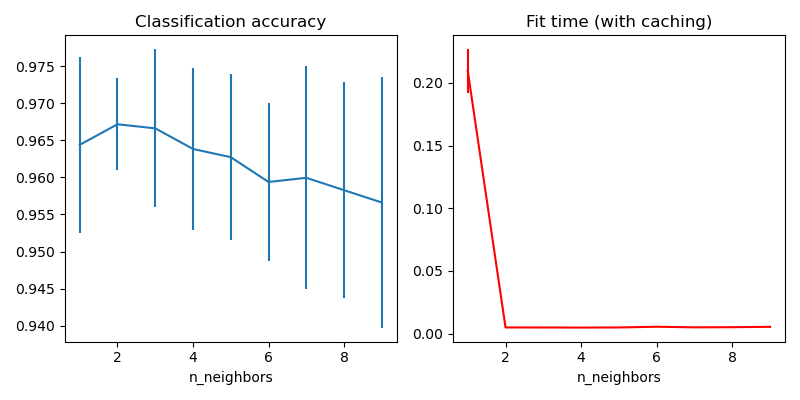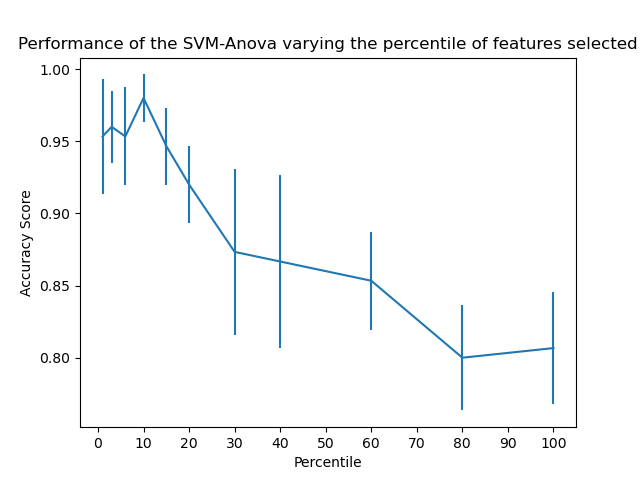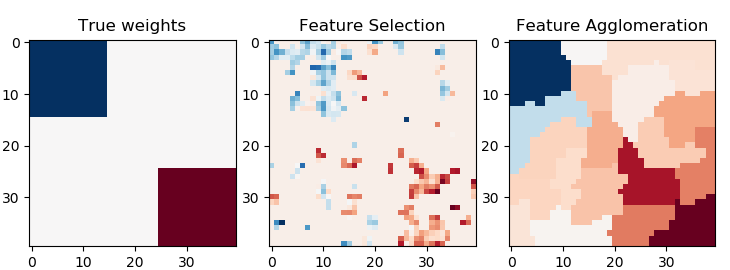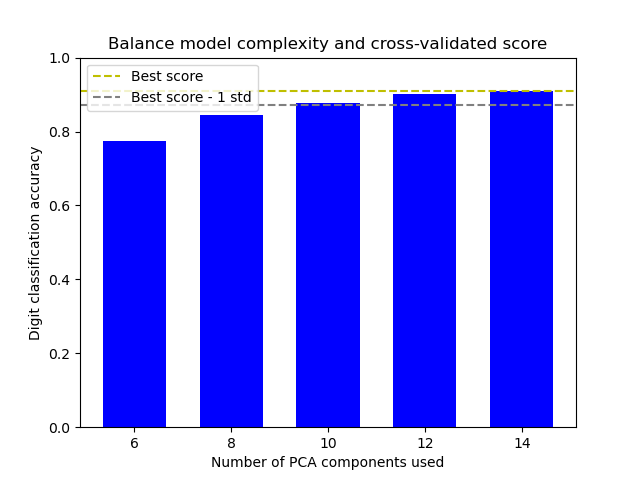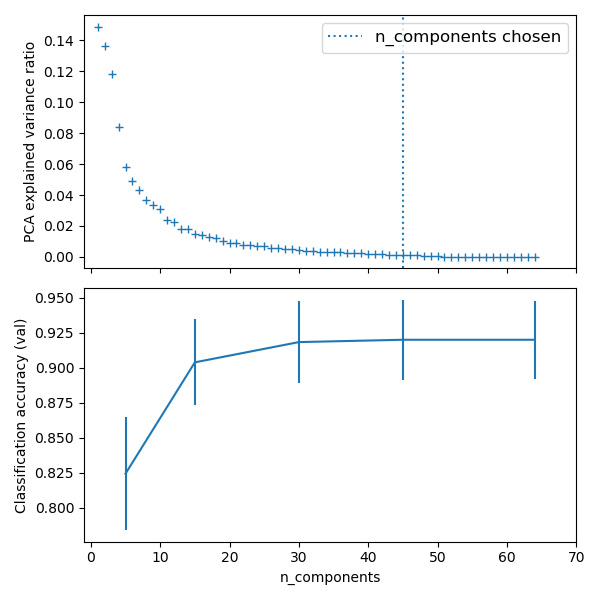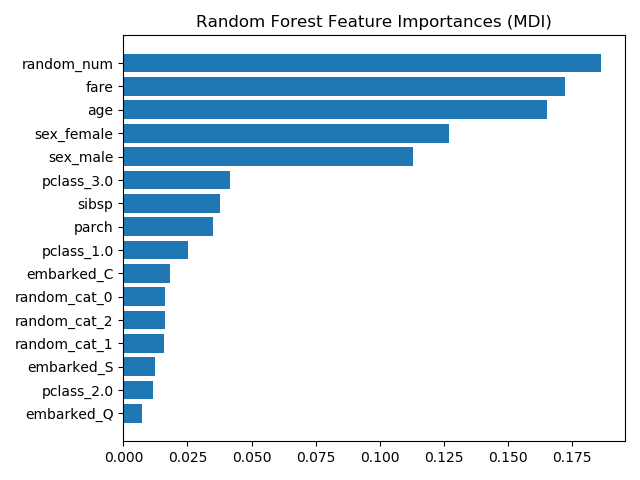# sklearn.pipeline.Pipeline¶

class sklearn.pipeline.Pipeline(steps, *, memory=None, verbose=False)

[源码]

0.5版的新功能。

steps list
(命名，转换）元组（实现拟合/转换）的列表，按照它们被链接的顺序，最后一个对象是估计器。
memory str or object with the joblib.Memory interface, default=None

verbose bool, default=False

named_steps Bunch

sklearn.pipeline.make_pipeline 便利函数，简化了管道建设。

>>> from sklearn.svm import SVC>>> from sklearn.preprocessing import StandardScaler>>> from sklearn.datasets import make_classification>>> from sklearn.model_selection import train_test_split>>> from sklearn.pipeline import Pipeline>>> X, y = make_classification(random_state=0)>>> X_train, X_test, y_train, y_test = train_test_split(X, y,...                                                     random_state=0)>>> pipe = Pipeline([('scaler', StandardScaler()), ('svc', SVC())])>>> # The pipeline can be used as any other estimator>>> # and avoids leaking the test set into the train set>>> pipe.fit(X_train, y_train)Pipeline(steps=[('scaler', StandardScaler()), ('svc', SVC())])>>> pipe.score(X_test, y_test)0.88

decision_function(X) 应用transforms，和最后一个估计器的decision_function
fit(X[, y]) 拟合模型
fit_predict(X[, y]) 在transforms后应用管道中最后一个step的fit_predict
fit_transform(X[, y]) 拟合模型并用最后一个估计器进行转换
get_params([deep]) 获取这个估计器的参数
predict(X, **predict_params) 将transforms应用于数据，并使用最后一个估计器进行预测
predict_log_proba(X) 应用transforms，和最后一个估计器的predict_log_proba
predict_proba(X) 应用transforms，和最后一个估计器的predict_proba
score(X[, y, sample_weight]) 应用transforms，和最后一个估计器的score
score_samples(X) 应用transforms，和最后一个估计器的score_samples
set_params(**kwargs) 为这个估计器设置参数
__init__(steps, *, memory=None, verbose=False)

[源码]

decision_function(X)

[源码]

X iterable

y_score array-like of shape (n_samples, n_classes)
fit(X, y=None, **fit_params)

[源码]

X iterable

y iterable, default=None

**fit_params dict of string -> object

self Pipeline

fit_predict(X, y=None, **fit_params)

[源码]

X iterable

y iterable, default=None

**fit_params dict of string -> object

y_pred array-like
fit_transform(X, y=None, **fit_params)

[源码]

X iterable

y iterable, default=None

**fit_params dict of string -> object

Xt array-like of shape (n_samples, n_transformed_features)

get_params(deep=True)

[源码]

deep bool, default=True

params mapping of string to any

property inverse_transform

Xt array-like of shape (n_samples, n_transformed_features)

Xt array-like of shape (n_samples, n_features)
predict(X, **predict_params)

[源码]

X iterable

**predict_params dict of string -> object

0.20版中的新功能。

y_pred array-like
predict_log_proba(X)

[源码]

X iterable

y_score array-like of shape (n_samples, n_classes)
predict_proba(X)

[源码]

X iterable

y_proba array-like of shape (n_samples, n_classes)
score(X, y=None, sample_weight=None)

[源码]

X iterable

y iterable, default=None

sample_weight array-like, default=None

score float
score_samples(X)

[源码]

X iterable

y_score ndarray of shape (n_samples,)
set_params(**kwargs)

[源码]

self
property transform

X iterable

Xt array-like of shape (n_samples, n_transformed_features)

## sklearn.pipeline.Pipeline使用示例¶